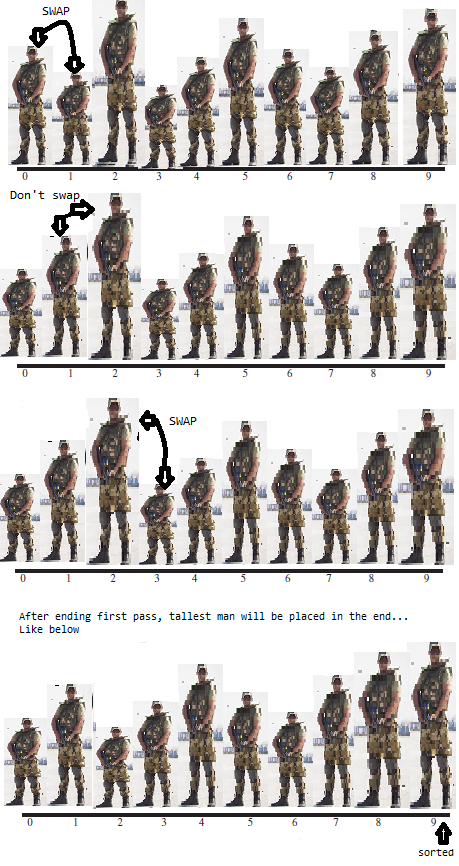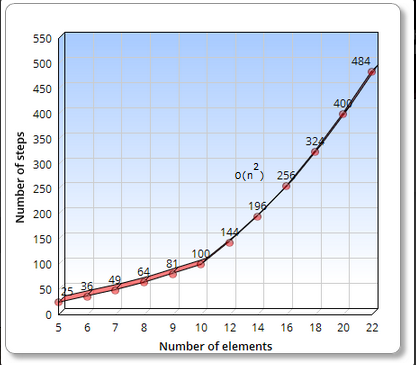### Bubble Sort in java

Contents of page >
• Diagram of bubble sort in java.
• Logic explanation of Bubble Sort in java >
• Full Program/SourceCode/Example of Bubble Sort in java >
• Complexity of Bubble Sort in java >
• Complexity of Bubble Sort in graph >

Bubble Sort is the simplest way of sorting the array. Complexity offered by bubble sort is O(n2).
We can use Selection Sort, Insertion Sort, merge Sort, quick Sort which may offer better time complexity.

Diagram of bubble sort in java >
Let’s see diagram below where we are going to arrange these people in ascending order of their  heights using Bubble Sort.Logic explanation of Bubble Sort in java >

Start comparing elements

If element in the left is taller as compared to element on immediate right, swap them.
If element in the left is smaller as compared to element on immediate right, don’t swap them.

Than, Move one position right.

Continue this process until you reach the right end. You will end up placing tallest person on extreme right.

After first pass, you would have made N-1 comparisons and
somewhere between 0 and N-1 swaps, depending on the initial arrangement of
the mens. The item at the end of the array is tallest(sorted) and we need not to move that element.

Now you go back to starting point and start another pass from the left end of the line. Again,
you go toward the right, following the above process of comparing and swapping. This time you can stop at position N-2 as last position was already sorted in first pass.

Continue this process until all the men’s become sorted.

Full Program/SourceCode/Example of Bubble Sort in java >
 /** * This class holds reference of array, * which has to be sorted. * And performs Bubble sorting in java */ class BubbleSortArray {       private int[] ar;    private int index; //holds current position of array, by default it is initialized with 0       /**    * Constructor for initializing Array    */    public BubbleSortArray(int maxSize) // constructor    {           ar = new int[maxSize]; // creation of array           index = 0;    }    /**    * This method inserts elements in Array.    */    public void insert(int value) {           ar[index++] = value; // increment the current index    }    /**    * This method Sort Array using Bubble Sort    */    public void bubbleSort(){           int outer, innner;           for (outer = index - 1; outer > 0; outer--) // outer loop (in backward direction)                  for (innner = 0; innner < outer; innner++)// inner loop (in forward direction)                        if (ar[innner] > ar[innner + 1]) // out of order?                               swap(innner, innner + 1); // swap them    } //End bubble sort method       /**    * This method swaps two elements in Array    */    private void swap(int pos1, int pos2) {           int temp = ar[pos1];           ar[pos1] = ar[pos2];           ar[pos2] = temp;    }       /**    * This method Display Array    */    public void display() {           for (int i = 0; i < index; i++)                  System.out.print(ar[i] + " ");           System.out.println("");    } } /** * Main class - Which will call BubbleSorting class */ public class BubbleSortingAlgorithm {    public static void main(String[] args) {                     int maxSize = 10; // initial size of Array                     BubbleSortArray bubbleSortArray =                        new BubbleSortArray(maxSize); // creation of array                     bubbleSortArray.insert(21);           bubbleSortArray.insert(1);           bubbleSortArray.insert(31);           bubbleSortArray.insert(51);           bubbleSortArray.insert(41);           bubbleSortArray.insert(91);           bubbleSortArray.insert(61);           bubbleSortArray.insert(32);           bubbleSortArray.insert(36);           bubbleSortArray.insert(93);              System.out.print("Display Array elements before bubble sorting: ");           bubbleSortArray.display(); // display Array elements before sorting                     bubbleSortArray.bubbleSort(); // bubble sort the array                     System.out.print("Display Array elements after bubble sorting: ");           bubbleSortArray.display(); // display Array elements after sorting    } } /** OUTPUT Display Array elements before bubble sorting: 21 1 31 51 41 91 61 32 36 93 Display Array elements after bubble sorting: 1 21 31 32 36 41 51 61 91 93 */

So, we wrote program to do Bubble Sort of array in java.

Complexity of Bubble Sort in java >
Best,average and worst case >

Best Case :    O(n2)
Average Case :  O(n2).
Worst Case :      The worst case too is O(n2), even though the code inside the if statement will not get executed in this case. The quadratic complexity is due to the fact that the two for loops will execute completely in all the three cases irrespective of the content of the list.

>No. of comparisons : O(n2) in best/average/worst case
>No. of swaps : O(n2) in worst case &
0 in best case(When array is already sorted).

Explanation of Bubble Sort in java>

Total number of comparisons in first pass=N-1 comparisons
Total number of comparisons in first pass=N-2 comparisons
Total number of comparisons in first pass=N-3 comparisons
and so on…

So we can calculate complexity by using following formula. The formula for the sum of such a
series is
(N–1) + (N–2) + (N–3) + ... + 1 = N*(N–1)/2
N*(N–1)/2 is 45 (10*9/2) when N is 10.

Sometimes swaps are less than comparisons because two elements are swapped only
if they need to be. If the data is random, a swap is necessary made about half the time, so
there will be about n2⁄4 swaps. (But in the worst case, when array is inversely sorted, a swap is made with every comparison.)

Both swaps and comparisons are proportional to n2. Therefore we can say that bubble sort runs in O(n2) time.

Also, in bubble sort we see outer loop nested within inner loop, you can find out that an algorithm
runs in O(n2) time.
The outer loop executes N times, and the inner loop also executes N times for each cycle of the outer loop. This means we’re doing approximately n*n or n2 times.

Complexity of Bubble Sort in graph >Summary >
So in this data structure tutorial we learned diagram of bubble sort in java. Logic explanation of Bubble Sort in java. Full Program/Example of Bubble Sort in java. Complexity of Bubble Sort in java. Complexity of Bubble Sort in graph.

Having any doubt? or you you liked the tutorial! Please comment in below section.

1) Sorting in java
2) Searching in java